### RSLFake Subsurface Effects

#### Introduction

Some objects, particularly those made of glass, can appear to have a rich sub-surface texture - figure 1. This tutorial demonstrates a shading technique that mimics sub-surface textures without the need to use ray tracing. The shaders on this page rely on a trick that strickly speaking should not be allowed by the RenderMan shading language, namely, allowing a surface shader to assign a value to point `P`. In theory only displacement shaders are allowed to change a micro-polygons `P` value.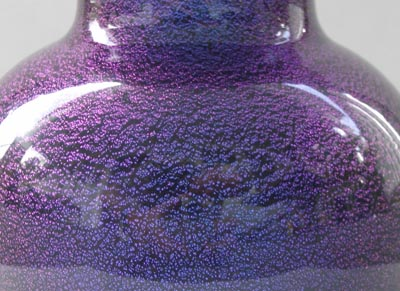Figure 1

The reflections from the surface of the vase shown in figure 1 appear to be smooth and glossy. However, the small scale bumps appear to unmistakenly "belong" to a layer of material that we interpret as beneath the surface of the glass. To reproduce this visual effect the shader in listing 2 makes two sets of lighting calculations sandwiched around a "temporary" displacement.

1. save copies of the true surface point `P` and normal `N`,
2. make a lighting calculation that includes environment mapping,
3. displace the suface point and recalculate the normal,
4. make another set of lighting calculations,
5. restore the "old" surface point and normal,
6. combine the two sets of lighting values.

#### Lighting Components

 ```color plastic(normal norm; vector incident; float ka, kd, ks, roughness; color hilitecolor) { normal n = normalize(norm); vector i = normalize(incident); normal nf = faceforward(n, i); color amb = ka * ambient(); color dif = kd * diffuse(nf); color spc = ks * specular(nf, -i, roughness) * hilitecolor; return (amb + dif + spc); } color envmapping(normal norm; vector incident; string mapname; string coordname; float kenv) { color envcolor = 0; if(mapname != "") { normal n = normalize(norm); vector i = normalize(incident); normal nf = faceforward(n, i); vector R = reflect(i, nf); R = transform(coordname, R); envcolor = environment(mapname, R) * kenv; } return envcolor; }```

The basic implementation of the shader is shown in listing 2. Listing 3 demonstrates the use of the shader in a rib file.

Listing 2

 ```#include "ShadingUtils.h" surface subsurf1(float Ka = 1, /* response to ambient */ Kd = 0.5, /* basic brightness */ Ks = 0.7, /* hilite brightness */ roughness = 0.1,/* surface smoothness */ subKa = 1, /* ditto sub-surf */ subKd = 0.5, /* ditto sub-surf */ subKs = 0.7, /* ditto sub-surf */ Km = 0, /* sub-surf bumpiness */ Kenv = 0, /* environ contribution */ Ktop = 0.5, /* scales the top color */ Ksub = 0.5; /* scales the sub color */ color hicolor = 1, /* top hilite color */ locolor = 1, /* sub-surf hilite color */ subCs = 1; /* sub-surf color */ string envname = "", envspace = "world") { // 1 Save copies of the "true" surface point oldP = P; normal oldN = N; Oi = Os; // 2 Obtain the environment lighting and the "top" layer color color envcolor = envmapping(N, I, envname, envspace, Kenv); color topcolor = plastic(N, I, Ka, Kd, Ks, roughness, hicolor) * Ktop; // 3 Apply a displacemnt and calculate a "fake" normal float hump = noise(s * 100, t * 250); P = P - hump * normalize(N) * Km; N = calculatenormal(P); // 4 Repeat the lighting (without environment mapping) with // the fake normal color sublight = plastic(N, I, subKa, subKd, subKs, roughness, locolor) * Ksub; color subcolor = sublight * subCs; // 5 Restore the true surface values P = oldP; N = oldN; // 6 Combine the top and sub-surface lighting Ci = Oi * Cs * (envcolor + topcolor + subcolor); } ```

#### Reference Renders

The next set of images provide a visual break-down of the output of the shader. The "trick" to this technique is producing a believable displacement. Figure 4 shows the effect of trying to procedurally produce high frequency displacements. It would have been better had the displacements been generated from a displacement map. How that can be achieved is left as a problem for the reader to solve.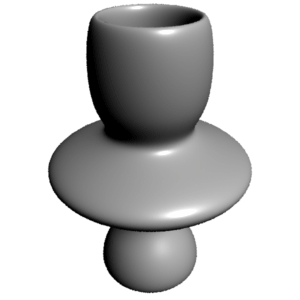Figure 2 - True surface geometry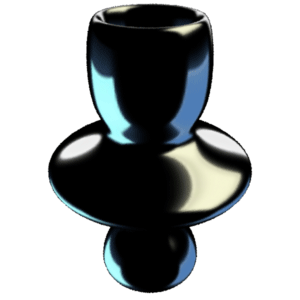Figure 3 - Environment map only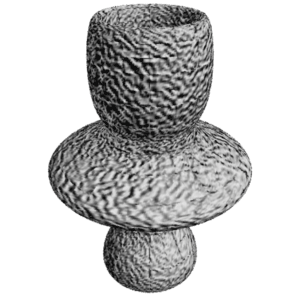Figure 4 - Displacements only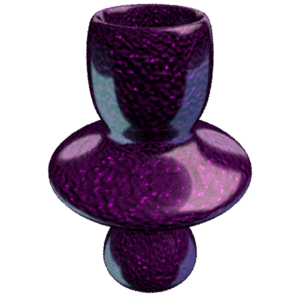Figure 5 - Overall effect

Listing 3

 ```Display "subsurf1" "framebuffer" "rgb" Format 400 400 1 Projection "perspective" "fov" 40 ShadingRate 2 Translate 0 -1.5 20 Rotate -30 1 0 0 Rotate 140 0 1 0 Scale 1 1 -1 WorldBegin TransformBegin # Orientate the environment map. Rotate 180 1 0 0 CoordinateSystem "_world" TransformEnd LightSource "distantlight" 1 "intensity" 1.5 "from" [1 4 5] "to" [0 0 0] TransformBegin Attribute "bound" "displacement" [0.1] Surface "subsurf1" "Km" 0.5 "Kd" 0.0 "Ks" 1 "subKd" 0.9 "subKs" 1 "Ktop" 0.2 "Ksub" 0.5 "Kenv" 0.5 "locolor" [1 1 1] "envname" ["env.tx"] "envspace" ["_world"] ReadArchive "vase.rib" TransformEnd WorldEnd```